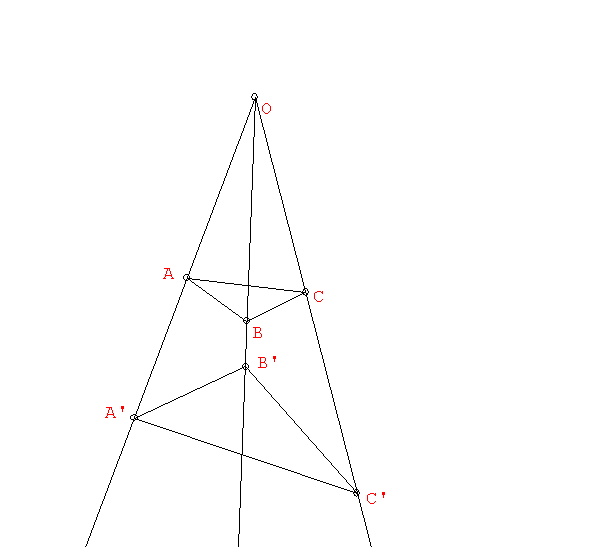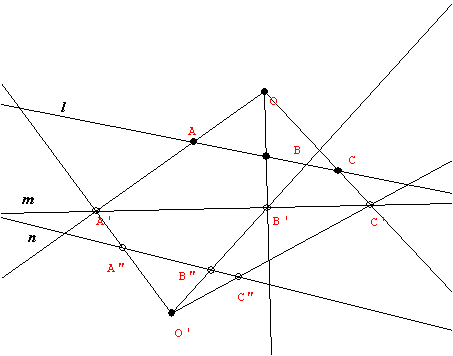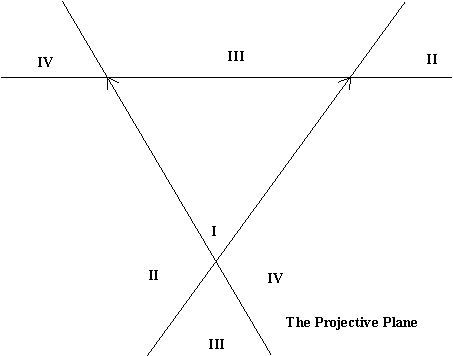Tuesday,  May 4

Projective geometry: The study of properties of figures that are related by projections... perspectivities and projectivities.

Examples: The projection of a line is a line. The point of intersection of two lines will project to the point of intersection of the projected lines.

Desargues' Theorem in Space:

We define a perspective relation:
Two points P and P' are perspectively related by the center O if O is on the line PP" . Two triangles ABC and A'B'C' are perspectively related by the center O if O is on the lines AA', BB', and CC'.Another aspect of Projection: Desargues' Theorem in 3-space and the plane.

Review:
Perpective between planes in Space!
How to see the Infinite using Projective geometry.

Seeing the horizon on a plane.... a line of ideal/infinite points.
How parallel lines "meet" on the horizon.

Use Rope and lines on floor with an image plane to see parallels meet --- where is the horizon?

Using coordinates to draw in perspective.

Activity: Creating coordinates in the projective plane and using them to transfer a circle to the projective plane as an ellipse.What do the conics look like in a perspective drawing or in the projective plane?

What is projection for Flatland?

Center of projection/ perspective.
[ compare with similarity].
Correspondences:  Activity:
Circle to line and line to Circle!
How to see the Infinite using Projective geometry.

Line to Line
Perspective and projective relations between points on lines.Activity on Perspective and Projective relations between points on lines.

Equations for conics in coordinate geometry.Equations for conics in coordinate geometry.
Using coordinates to draw in perspective.

What do the conic look like in a perspective drawing or in the projective plane?Connecting the projective plane with the flattened surface for the projective plane.

What is projection for Flatland?

Center of projection/ perspective.
[ compare with similarity].
Correspondences:  Activity:Circle to Line:

How to see the Infinite using Projective geometry.

Circle to line and line to Circle!
Line to Line
Perspective and projective relations between points on lines.Activity on Perspective and Projective relations between points on lines.

Perpective between planes in Space!

Seeing the horizon on a plane.... a line of ideal/infinite points.

How parallel lines "meet" on the horizon.
What is the Projective Plane?How do we see curves in perspective, drawn in the Projective Plane?

Desargues' Theorem.